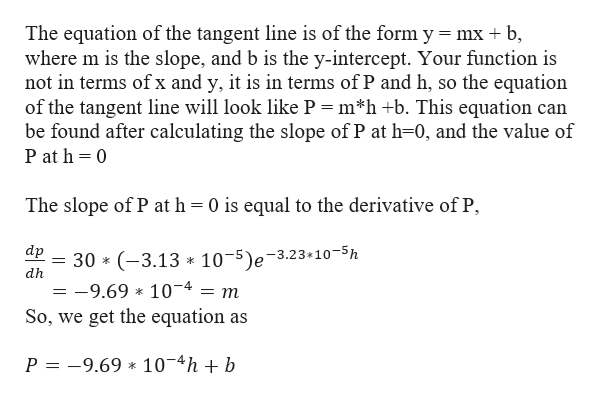# Air pressure at sea level is 30 inches of mercury. At an altitude of h feet above sea level, theair pressure, P, in inches of mercury, is given byP(h)= 30e(-3.23*10^-5h))(a)Find the local linearization of P(h) near h= 0.(b)A common 'rule of thumb' used by travelers is that air pressure drops about 1 inch for every 1000-foot increase in height above the sea level. Explain why this 'rule of thumb' gives a reasonable method of estimation.(c)Does the local linearization found in part (a) give overestimates or underestimates of the exact air pressure? Explain why.(d)Does the 'rule of thumb' from part (b) give overestimates or underestimates of the exact air pressure? Explain why

Question
550 views
Air pressure at sea level is 30 inches of mercury. At an altitude of h feet above sea level, the
air pressure, P, in inches of mercury, is given by
P(h)= 30e(-3.23*10^-5h))
(a)
Find the local linearization of P(h) near h= 0.
(b)
A common 'rule of thumb' used by travelers is that air pressure drops about 1 inch for every 1000-foot increase in height above the sea level. Explain why this 'rule of thumb' gives a reasonable method of estimation.
(c)
Does the local linearization found in part (a) give overestimates or underestimates of the exact air pressure? Explain why.
(d)
Does the 'rule of thumb' from part (b) give overestimates or underestimates of the exact air pressure? Explain why
check_circle

star
star
star
star
star
1 Rating
Step 1

Given function,

Step 2

By the definition of a tangent line is defined as a line passing through a given point of a function whose slope is equal to the derivative of the function at that pointhelp_outlineImage TranscriptioncloseThe equation of the tangent line is of the form y = mx b where m is the slope, and b is the y-intercept. Your function is not in terms of x and y, it is in terms of P and h, so the equation of the tangent line will look like P = m*h +b. This equation can be found after calculating the slope of P at h-0, and the value of P at h 0 The slope of P at h = 0 is equal to the derivative of P (-3.13 10-5)e-3.23 10-5h -9.69 104 So, we get the equation as dp 30 dh m P =-9.69 10-4hb * fullscreen
Step 3

we need to find b, the y intercept, to completely solve for the tangent ...

### Want to see the full answer?

See Solution

#### Want to see this answer and more?

Solutions are written by subject experts who are available 24/7. Questions are typically answered within 1 hour.*

See Solution
*Response times may vary by subject and question.
Tagged in

### Derivative